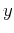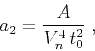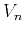Nonhyperbolic reflection moveout of-waves: An overview and comparison of reasonsNext: ANISOTROPY VERSUS LATERAL HETEROGENEITY Up: CURVILINEAR REFLECTOR Previous: Curved reflector beneath isotropic

## Curved reflector beneath homogeneous VTI medium

For a dipping curved reflector in a homogeneous VTI medium, the ray trajectories of the incident and reflected waves are straight, but the location of the reflection point is no longer controlled by the isotropic laws. To obtain analytic expressions in this model, we use the theoremthat connects the derivatives of the common-midpoint traveltime with the derivatives of the one-way traveltimes for an imaginary wave originating at the reflection point of the zero-offset ray. This theorem, introduced for the second-order derivatives by Chernjak and Gritsenko (1979), is usually called the normal incidence point (NIP) theorem (Hubral, 1983; Hubral and Krey, 1980). Although the original proof did not address anisotropy, it is applicable to anisotropic media because it is based on the fundamental Fermat's principle. The normal incidence'' point in anisotropic media is the point of incidence for the zero-offset ray (which is, in general, not normal to the reflector). In Appendix A, we review the NIP theorem, as well as its extension to the high-order traveltime derivatives (Fomel, 1994).

Two important equations derived in Appendix A are:(66)(67)

whereis the one-way traveltime of the direct wave propagating from the reflection pointto the pointat the surface. All derivatives in equations (66) and (67) are evaluated at the zero-offset ray. Both equations are based solely on Fermat's principle and, therefore, remain valid in any type of media for reflectors of an arbitrary shape, assuming that the traveltimes possess the required order of smoothness. It is especially convenient to use equations (66) and (67) in homogeneous media, where the direct traveltimecan be expressed explicitly.

To apply equations (66) and (67) in VTI media, we need to start with tracing the zero-offset ray. According to Fermat's principle, the ray trajectory must correspond to an extremum of the traveltime. For the zero-offset ray, this simply means that the one-way traveltimesatisfies the equation(68)

where(69)

Here, the functiondescribes the reflector shape, andis the ray angle given by the trigonometric relationship (Figure 5)(70)

Substituting approximate equation (5) for the group velocityinto equation (69) and linearizing it with respect to the anisotropic parametersand, we can solve equation (68) for, obtaining(71)

or, in terms of,(72)

whereis the local dip of the reflector at the reflection point. Equation (72) shows that, in VTI media, the angleof the zero-offset ray differs from the reflector dip(Figure 5). As one might expect, the relative difference is approximately linear in Thomsen anisotropic parameters.nmoray
Figure 5.
Zero-offset reflection from a curved reflector beneath a VTI medium (a scheme). Note that the ray angleis not equal to the local reflector dip.Now we can apply equation (66) to evaluate the second term of the Taylor series expansion (22) for a curved reflector. The linearization in anisotropic parameters leads to the expression(73)

which is equivalent to that derived by Tsvankin (1995). As in isotropic media, the normal-moveout velocity does not depend on the reflector curvature. Its dip dependence, however, is an important indicator of anisotropy, especially in areas of conflicting dips (Alkhalifah and Tsvankin, 1995).

Finally, using equation (67), we determine the third coefficient of the Taylor series. After linearization in anisotropic parameters and lengthy algebra, the result takes the form(74)

where(75)

and the coefficientis defined by equation (58). For zero curvature (a plane reflector), and the only term remaining in equation (75) is(76)

If the reflector is curved, we can rewrite the isotropic equation (63) in the form(77)

where the normal-moveout velocityand the quantityare given by equations (73) and (75), respectively. Equation (77) approximates the nonhyperbolic moveout in homogeneous VTI media above a curved reflector. For small curvature, the accuracy of this equation at finite offsets can be increased by modifying the denominator in the quartic term similarly to that done by Grechka and Tsvankin (1998) for VTI media.Nonhyperbolic reflection moveout of-waves: An overview and comparison of reasonsNext: ANISOTROPY VERSUS LATERAL HETEROGENEITY Up: CURVILINEAR REFLECTOR Previous: Curved reflector beneath isotropic

2014-01-27